Custom Search
If you want to discuss more on this or other issues related to Physics, feel free to leave a message on my Facebook page.

## Tuesday, November 24, 2009

### Speed and velocity

After distance and displacement that we have seen earlier, I am now going to talk about speed and velocity. They are quite different and to understand them it is important to understand clearly the difference distance and displacement before going forward.

Speed

Speed is a scalar quantity and the unit is the metre per second (m s-1)

A boy moves from point A and walks a distance of 50 m to the point B in a time of 20 s.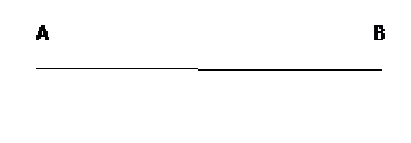Firstly what is the distance travelled in 1 second?

Yes the boy would walk a distance of 2.5 m in a time of 1 second.

The distance travelled in a time of 1 second is called the speed.

The equation to calculate the speed is shown below.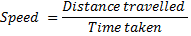Can you determine the speed of the boy?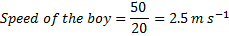Hence whenever speed is calculated the distance should be used.

Velocity

Velocity is a vector quantity and the unit is also the metre per second(m s-1)

Since it is a vector quantity then when it is determined direction is important and hence displacement should be used.

A boy moves from point A to point B performing a displacement of 50 m towards the right as shown in the diagram below.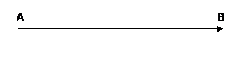The following equation is used to determine the velocity.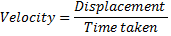Hence the velocity of the boy can be calculated as shown below.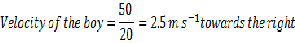Please note the difference between the two. For the speed the distance travelled is used while for the velocity the displacement is used.